5.2 The Distributive Property

# Learning Objectives

• Distribute the number outside of the parentheses with both of the values inside the parentheses and find the sum of those numbers.
• Show that distributive property yields the same answer as PEMDAS.

# Introduction

The Omni Theater DilemmaThree days before the trip, Mrs. Sanchez comes running up to Kyle.

She has discovered that there is an Omni Theater at the Science Museum and they are showing a film on the Rainforest. Kyle is thrilled! He loves the Omni Theater.

However, the problem is that it will cost an additional two dollars for each of the students to attend the showing. The chaperones can all go for free.

“Can you work this out?” Mrs. Sanchez asks Kyle. “There are fifty dollars in our class account plus the money that you have already collected from the students. How much money total will we need to go to both the museum and the Omni Theater?”

“I will handle it,” Kyle says. “I think we have enough money for everything. Let me figure it out.”

Mrs. Sanchez smiles and goes back to work.

Kyle takes out a piece of paper and a pencil. He writes down the following information.

22 students with an admission price of $8.95 22 students with an Omni Theater price of$2.00

Ah! Kyle remembers that he can use parentheses to help him out with this problem. Here is what he finally writes.

22(8.95 + 2.00)

Kyle stops. He knows that there is a way to solve this with the Distributive Property, but he can’t remember exactly what to do.

This is where you come in.

In this lesson, you will learn how to use the Distributive Property to solve problems where there is a sum being multiplied by a number.

By the end of the lesson, you will be able to help Kyle with his problem.

# Guided Learning

## Write Numerical Expressions for the Product of a Number and a Sum

We know that a numerical expression is a statement that has more than one operation in it.

When we write an expression, we want it to illustrate mathematical information in a correct way.

We can write expressions that contain all kinds of combinations of operations. Today, we are going to learn about how to write an expression that involves the product of a number and a sum.

How do we write an expression that involves the product of a number and a sum?

The first thing that we need to do is to decipher these words so that we can understand what we are actually talking about.

The product of a number – we know that product means multiplication. We are going to be multiplying this number.

And a sum – the word sum means addition. We are going to have a sum here. That means that we will have two numbers that are being added together.

The tricky thing about this wording is that it talks about the product of a number AND a sum. That means that we are going to be multiplying a number by an ENTIRE sum.We can figure out what this looks like by first taking a number.

Let’s use 5.

Then we take a sum.

Let’s use 4 + 3.

Now because we want to multiply the number times the sum, we need to put the sum into parentheses.

Here is our answer.

5(4 + 3)

This is a numerical expression for the information.

Let’s look at an example.

### Example A

Write a numerical expression for the product of 2 times the sum of 3 and 4.

Here we know that two is going to be outside the parentheses, meaning “the product of 2”

3 plus 4 will be inside the parentheses-this is the sum.

Here is our expression.

Our answer is 2(3 + 4).

# Guided Practice

Try writing a few of these on your own.

1. The product of three and the sum of four plus five.
2. The product of four and the sum of six plus seven.
3. The product of nine and the sum of one plus eight.Take a minute to check your work with a friend. Did you write the expression correctly?

Solutions:

1. 3(4 + 5)
2. 4(6 +7)
3. 9(1 +8)

## Identify and Apply the Distributive Property to Evaluate Numerical Expressions

We just finished learning how to write a numerical expression that has the product of a number and a sum. Now we are going to work on evaluating those expressions.

What does the word “evaluate” mean?

When we evaluate an expression, we figure out the value of that expression or the quantity of the expression.

When we evaluate expressions that have a product and a sum, we use a property called the Distributive Property.

What is the Distributive Property?

The Distributive Property is a property that is a true statement about how to multiply a number with a sum. Multiply the number outside the parentheses with each number inside the parentheses. Then figure out the sum of those products.

In other words, we distribute the number outside the parentheses with both of the values inside the parentheses and find the sum of those numbers.

Let’s see how this works.

### Example B

4(3 + 2)

To use the Distributive Property, we take the four and multiply it by both of the numbers inside the parentheses.

Then we find the sum of those products.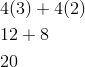Our answer is 20.

Here is another one.

### Example C

8(9 + 4)

Multiply the eight times both of the numbers inside the parentheses.

Then find the sum of the products.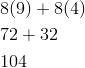Our answer is 104.

## Guided Practice

Now it is your turn. Evaluate these expressions using the Distributive Property. Show all your work.

1. 5(6 + 3)
2. 2(8 + 1)
3. 12(3 + 2)Now check your work with a peer.

Solutions:

1. (5 x 6) + (5 x 3) = (30) + (15) = 45
2. (2 x 8) + (2 x 1) = (16) + (2) = 18
3. (12 x 3) + (12 x 2) = (36) + (24) = 60

## Evaluate Products Using Mental Math

Some of you may have found that while the Distributive Property is useful, that sometimes it is easier to simply find the products by using mental math.

Some of you may have found that you did not need to write out the distribution of the number outside of the parentheses with the number inside of the parentheses to find the sum of the products.

The Distributive Property is a useful property, especially as you get into higher levels of mathematics like Algebra. There it is essential, but sometimes, you can use mental math to evaluate expressions.

Let’s look at this example.

### Example D

2(1 + 4)

Now this is an example where you could probably add and multiply in your head.

You know that you can add what is in parentheses first, so you add one and four and get five.

Then you can multiply five times two and get a product of 10.

Our answer is 10.

When you have larger numbers, you can always use the Distributive Property to evaluate an expression. When you have smaller numbers, you can use mental math.

## Guided Practice

Practice your mental math by evaluating these expressions.

1. 4(2 + 3)
2. 6(2 + 7)
3. 5(2 + 6)Take a minute to compare your answers with a neighbor’s. Were you able to complete the addition and multiplication without a piece of paper?

Solutions:

1. 4(5) = 20
2. 6(9) = 54
3. 5(8) = 40

## Apply the Distributive Property to Evaluate Formulas Using Decimal Quantities

We can also use and apply the Distributive Property when working with a formula. Let’s think about the formula for finding the area of a rectangle.

We know that the area of a rectangle can be found by using the formula: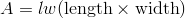However, for this example we have 2 areas, so we would multiply 12 times 4.5 and get an area of 54 square inches.        And we would multiple 7 times 4.5 and get an area of 31.5 square inches. Then we would add the two areas together, for a total area of 85.5 square inches.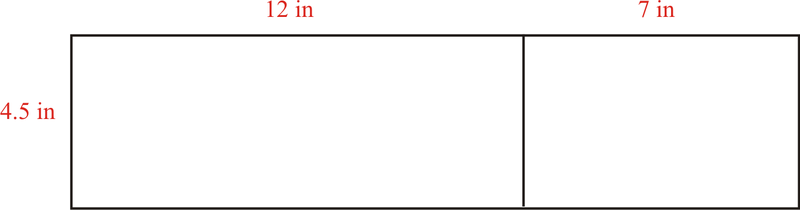How can we find the area of both of these rectangles without the added at the end?

You can see that they have the same width. The width is four and a half inches.

However, there are two lengths.

We need to find the product of a number and a sum.

Here is our expression.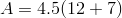Now we can use the Distributive Property to find the area of these two rectangles.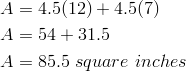Notice that we used what we have already learned about multiplying decimals and whole numbers with the Distributive Property.

When we distributed 4.5 with each length, we were able to find the sum of the products.

This gives us the area of the two rectangles.

## Guided Practice

Here is an example for you to try on your own.

1.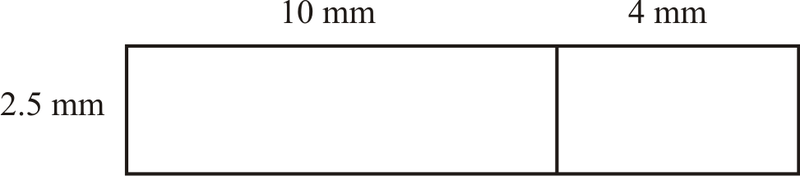Check your answer with a friend.

Solution:

1. 2.5 (10 + 4) = 2.5(10) + 2.5(4) = 25 + 10 = 35 mm

### Real Life Example Completed

The Omni Theater DilemmaThree days before the trip, Mrs. Sanchez comes running up to Kyle.

She has discovered that there is an Omni Theater at the Science Museum and they are showing a film on the Rainforest. Kyle is thrilled! He loves the Omni Theater.

However, the problem is that it will cost an additional two dollars for each of the students to attend the showing. The chaperones can all go for free.

“Can you work this out?” Mrs. Sanchez asks Kyle. “There are fifty dollars in our class account plus the money that you have already collected from the students. How much money total will we need to go to both the museum and the Omni Theater?”

“I will handle it,” Kyle says. “I think we have enough money for everything. Let me figure it out.”

Mrs. Sanchez smiles and goes back to work.

Kyle takes out a piece of paper and a pencil. He writes down the following information.

22 students with an admission price of $8.95 22 students with an Omni Theater price of$2.00

Ah! Kyle remembers that he can use parentheses to help him out with this problem. Here is what he finally writes.

22(8.95 + 2.00)

Kyle stops. He knows that there is a way to solve this with the Distributive Property, but he can’t remember exactly what to do.

First, let’s go back and underline all of the important information.

Now we can take the expression that Kyle wrote and use the Distributive Property to figure out the total amount of money needed for the trip.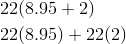Next, we can multiply 22 by 8.95.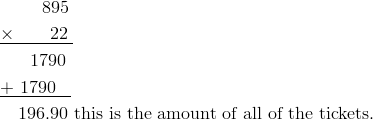Next, we complete the second part of the problem.

2(22) = 44

It will cost the students an additional $44.00 to attend the Omni Theater. The good news is that there is enough money in the student account to help cover the additional costs. There are fifty dollars in the account and the class only needs$44.00 to help cover the costs.

The total amount of money needed is \$240.90.

Kyle gives his information to Mrs. Sanchez and she is thrilled!

Now the students are off to the Science Museum and the Omni Theater!

# Review

• Numerical expression is a statement that has more than one operation in it.
• Product is the answer to a multiplication problem.
• Sum is the answer to an addition problem.

Evaluate

To figure out the value of an expression.

The Distributive Property

A property that is a true statement about how to multiply a number with a sum. Multiply the number outside the parentheses with each number inside the parentheses. Then figure out the sum of those products.

# Video Resources

Distributive Property Video

Distributive Property 2 Video